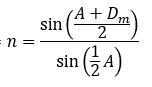# Index of Refraction Uncertainty Problem

Homework Statement:
Trying to find the uncertainty in individual measurements of the index of refraction of a prism
Relevant Equations:
n=sin⁡((A+D_m)/2)/sin⁡(1/2 A)A is the angle of refraction (~60 degrees) and Dm is the angle of minimum deviation that was different for each of the spectral lines associated to one of the six wavelengths measured.
The problem I'm having is when I use equations of propagation of error to find the uncertainty in these indices, the uncertainty ends up coming with units of degrees which I'm almost certain is correct as indices have no units.

An example trial is: A = 59.97 degrees and Dm = 45.6 degrees

Thank you.

haruspex
Homework Helper
Gold Member
Homework Statement: Trying to find the uncertainty in individual measurements of the index of refraction of a prism
Homework Equations: n=sin⁡((A+D_m)/2)/sin⁡(1/2 A)

View attachment 250256
A is the angle of refraction (~60 degrees) and Dm is the angle of minimum deviation that was different for each of the spectral lines associated to one of the six wavelengths measured.
The problem I'm having is when I use equations of propagation of error to find the uncertainty in these indices, the uncertainty ends up coming with units of degrees which I'm almost certain is correct as indices have no units.

An example trial is: A = 59.97 degrees and Dm = 45.6 degrees

Thank you.